# Average problems tricks | average arithmetic formulae | average short-cuts

•
•
•
•
•
•
•
•

We are going to talk about the very important arithmetic formulae on average, which are very helpful for all types of competitive exams.

# AVERAGE PROBLEM-SOLVING TRICKS1). If the number of the quantities and their sum is given, the average is given by =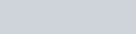2). If the no. of the quantities and its average is given then the sum of the quantities is =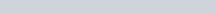3). If the sum and its average is given then the no. of the quantities is =4). If the average of A boys is x, and the average of B-girls is y, if all of them is put together, then the average is =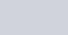5). If the average of A boys is x, and the average of B-boys out of A boys is y then the average of the rest of boys is =6). If the average of A objects is x if any object is removed from the list then average become y then the magnitude of the removed object is =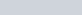7). If the average of A no. is a, if x is added or subtracted from each no. then the average becomes =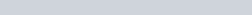8). If the average of A quantities is x, and if a new quantity is added then average become y, then the added new quantity is =9). If the average of A no. is x, then if each no. is multiplied or divided by y then, the average becomes =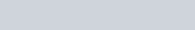10). If the average weight of A person is increased by x kg if one person of weight, y kg is replaced by a new person, then the weight of the new person is =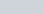*If the average is decreasing then the weight of the new person is =11). The average marks obtained by A candidates in a certain examination is m if the average marks of the passed candidate are n, and the fail candidate is o, then the no of candidates who passed the exam is =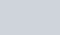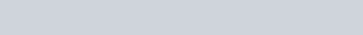12). If the average of n odd numbers is ‘a’, and the average of first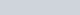number is ‘b’ if lastnumber is ‘c’ then theth no. is =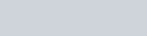13). If a batsman in n innings makes a score of x, and the average is increased by y, then average after n innings is =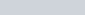14). If a batsman has an average of x runs after the completion of n innings. Then no of runs he has to make to raise his average to y is =15). If a person travels a distance with x km/h, and again travels the same distance with y km/h, then the average speed for the whole journey is =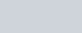* If half of the distance is traveled by x km/h and the other half distance is traveled by y km/h then the average for the whole journey is =* If person goes with x km/h and return with y km/h then average speed is =16). If a person travels three equal distances with x km/h, y km/h, and z km/h then the average speed for the whole journey is =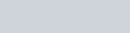17). If a person travels A km by x km/h, B km by y km/h, and C km by z km/h then the average speed for the whole journey is =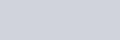18). A person travels Ath part of a distance with x km/h, Bth part of the distance with y km/h, and Cth part with z km/h then average speed for the whole journey is =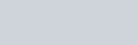* If Ath, Bth, and Cth part of the distance is given as A%, B%, and C% then formula change to the average speed of =19). The average value of all the members of a group is x if the first part of members has an average of y, and the average of the remaining parts of members is z and no. of members in the first part is n then no. of members is remaining part =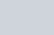* If n is the no. of members in the remaining part then the no. of members in the first part is =20). The average of first n natural number is =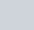21). The average of n consecutive number is the middle number

22). The average of even n consecutive numbers is the average of the middle two numbers.

* The average of two middle number is calculated as follows:
** In case of consecutive numbers-
Average = smaller middle number +0.5 or greater middle number -0.5

** In case of consecutive odd and consecutive even-
Average = smaller middle number +1 or greater middle number -1

23). The average of odd number from 1 to n, (where n is a natural odd number) is =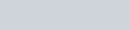24). The average of even natural number from 1 to n is =25). The average of the square of natural number till n is =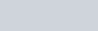26). The average of cubes of natural number till n is =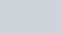27). The average of the first n consecutive even number is =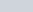28). The average of the first n consecutive odd number is =n

29). The average of squares of first n consecutive even number is =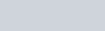30). The average of squares of even number till n is =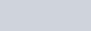31). The average of squares of consecutive odd number till n is =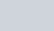32). The average of n numbers is A , and rechecking it is find that some of the numbers that is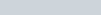are taken wrongly asthen the correct average is =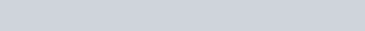33). The average of a series having a common difference 2 is =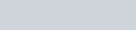34). If the average of n consecutive odd numbers is x, then the difference between the largest and smallest number is =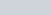35). If P distance is travelled by x km/h, Q distance with y km/h, R distance with z km/h, then average speed for whole journey is =36). The average weight of a group of X members is y if after entering or exiting of a member, the average weight become z, then the weight of entering or exiting person is =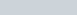37). The average weight of a group of x person is y when z person get enter/exit in the group, the average of the group become w, then the average of new entering/exiting persons is =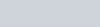38). In the group of x persons, if a t years old person is replaced by a new person, then the average is increased/ decreased by t1, then the age of a new person is =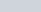39). The average of n multiple of any number is =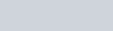For more such tricks articles stay tuned with Laws Of Nature